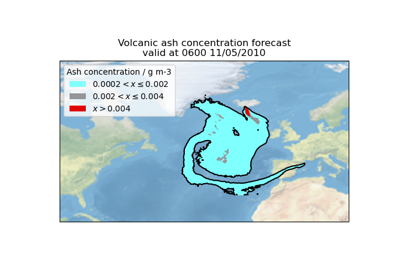# General#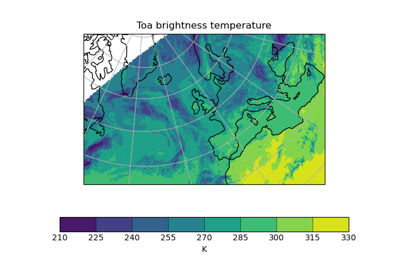Example of a Polar Stereographic Plot

Example of a Polar Stereographic Plot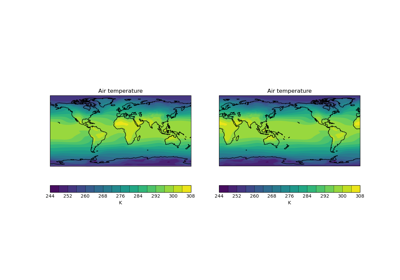Quickplot of a 2D Cube on a Map

Quickplot of a 2D Cube on a Map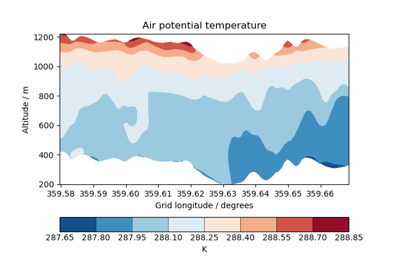Cross Section Plots

Cross Section Plots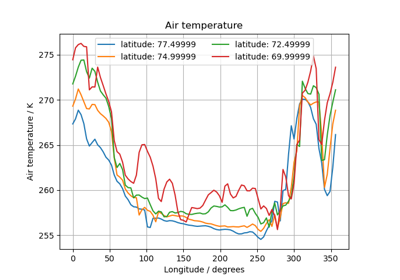Multi-Line Temperature Profile Plot

Multi-Line Temperature Profile Plot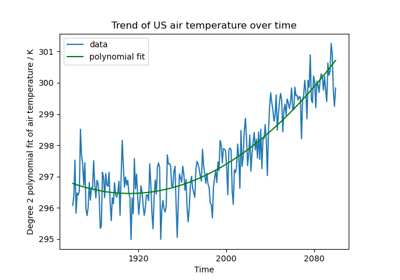Fitting a Polynomial

Fitting a Polynomial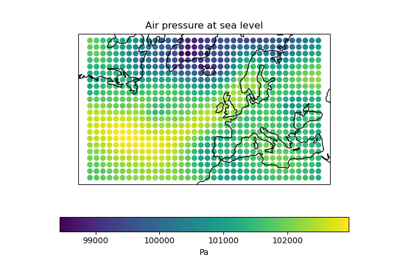Rotated Pole Mapping

Rotated Pole Mapping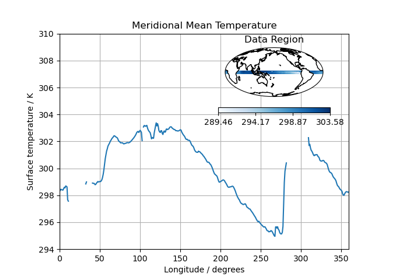Test Data Showing Inset Plots

Test Data Showing Inset Plots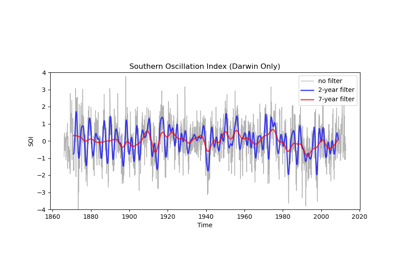Applying a Filter to a Time-Series

Applying a Filter to a Time-Series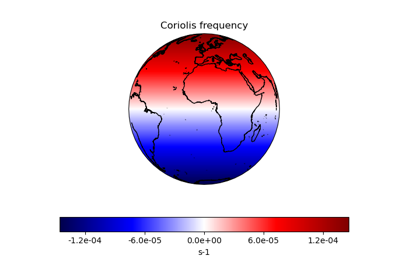Deriving the Coriolis Frequency Over the Globe

Deriving the Coriolis Frequency Over the Globe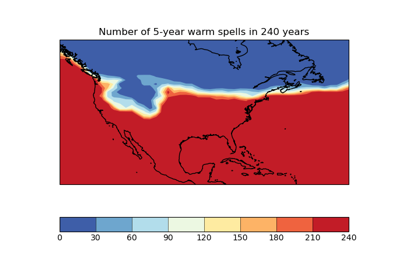Calculating a Custom Statistic

Calculating a Custom Statistic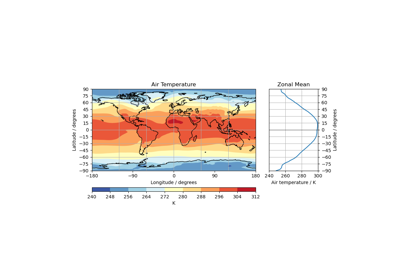Zonal Mean Diagram of Air Temperature

Zonal Mean Diagram of Air Temperature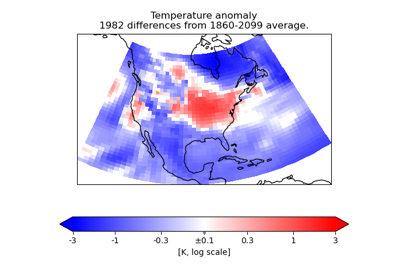Colouring Anomaly Data With Logarithmic Scaling

Colouring Anomaly Data With Logarithmic Scaling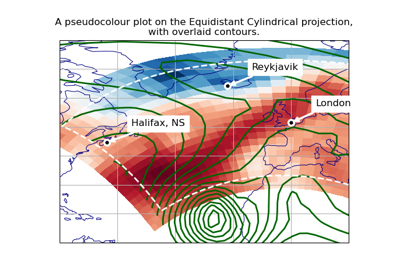Plotting in Different Projections

Plotting in Different Projections